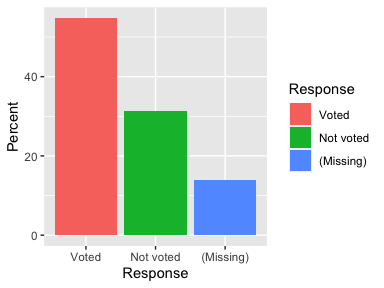toplines

library(pollster)
library(dplyr)
library(knitr)
library(ggplot2)

The default topline table comes with columns for response category, frequency count, percent, valid percent, and cumulative percent.

topline(df = illinois, variable = voter, weight = weight) %>%
kable()
Response Frequency Percent Valid Percent Cumulative Percent
Voted 56230937 54.76407 63.6809 63.6809
Not voted 32070164 31.23357 36.3191 100.0000
(Missing) 14377412 14.00236 NA NA

Because the output is a tibble, it’s simple to manipulate it in any way you want after creating it. Use dplyr::select to remove columns or dplyr::filter to remove rows. For convenience, the topline function also provides ways to do this within the function call. For example, the remove argument accepts a character vector of response values to be removed from the table after all statistics are calculated. This is especially useful for survey data with a “refused” category.

topline(df = illinois, variable = voter, weight = weight,
remove = c("(Missing)"), pct = FALSE) %>%
mutate(Frequency = prettyNum(Frequency, big.mark = ",")) %>%
kable(digits = 0)
Response Frequency Valid Percent Cumulative Percent
Voted 56,230,937 64 64
Not voted 32,070,164 36 100

Refer to the kableExtra package for lots of examples on how to format the appearance of these tables in either HTML or PDF latex formats. I recommend the vignettes “Create Awesome HTML Table with knitr::kable and kableExtra” and "Create Awesome PDF Table with knitr::kable and kableExtra.

Graphs

topline(df = illinois, variable = voter, weight = weight) %>%
ggplot(aes(Response, Percent, fill = Response)) +
geom_bar(stat = "identity")Margin of error

Get at topline table with the margin of error in a separate column using the moe_topline function. By default, a z-score of 1.96 (95% confidence interval is used). Supply your own desired z-score using the zscore argument.

moe_topline(df = illinois, variable = educ6, weight = weight)
#> # A tibble: 6 x 6
#>   Response Frequency Percent Valid Percent   MOE Cumulative Percent
#>   <fct>        <dbl>   <dbl>           <dbl> <dbl>                <dbl>
#> 1 LT HS    10770999.   10.5            10.5  0.326                 10.5
#> 2 HS       31409418.   30.6            30.6  0.490                 41.1
#> 3 Some Col 21745113.   21.2            21.2  0.435                 62.3
#> 4 AA        8249909.    8.03            8.03 0.289                 70.3
#> 5 BA       19937965.   19.4            19.4  0.421                 89.7
#> 6 Post-BA  10565110.   10.3            10.3  0.323                100

The margin of error is calculated including the design effect of the sample weights, using the following formula:

sqrt(design effect)*zscore*sqrt((pct*(1-pct))/(n-1))*100

The design effect is calculated using the formula length(weights)*sum(weights^2)/(sum(weights)^2).